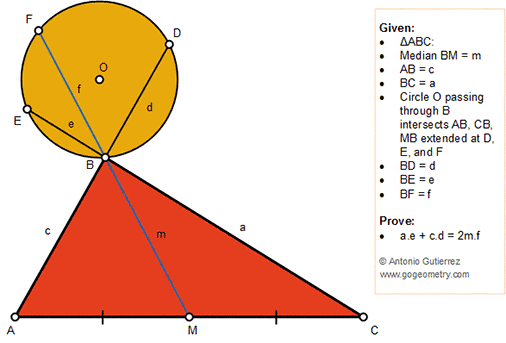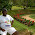Friday, August 31, 2018

Geometry Problem 1383: Triangle, Median, Circle, Chord, Secant, Sum, Product

Geometry Problem. Post your solution in the comment box below.
Level: Mathematics Education, High School, Honors Geometry, College.

Details: Click on the figure below.1.https://photos.app.goo.gl/xJDBQXw4WMGRvhSH9

Let ∠ (BFE)= u and ∠ (BFD)= v
Draw MA’ so that ∠ (BA’M)= ∠ (BFD)= v ..( see sketch)
MA’ cut BC at C’
Let BA’=c’ and BC’=a’
In cyclic quadrilateral EBDF we have angle B supplement to (u+v)
In triangle BA’C’ we have angle B supplement to angle ( v+ ∠ (BC’M)) so ∠ (BC’M)= u
Triangle BFD similar to BMA’ (case AA) => d/f= m/c’ => c.d/m.f= c/c’ ( 1)
Triangle BFE similar to BC’M => e/f= m/a’ => a.e/m.f= a/a’ … (2)
Draw AL //A’C’ and CN //A’C’ and note that ML=MN= x
In similar triangles BMC’ and BNC we have a/a’= BN/BM= 1+x/BM… (3)
Similarly c/c’= BL/BM= 1-x/BM…(4)
Add (3) to (4) we have a/a’+c/c’= 2
Replace value of a/a’ and c/c’ from (1) and (2) we have ae/mf+cd/mf= 2
Or ae+cd= 2mf

2.3.Let circle ADF cut BM at X and let circle CEF cut BM extended at Y

Let < XAC = x and let < XCA = y

< DAX = A - x = < DFX &
< ECX = B + y = < EFX

Considering triangle ABC, A + B = < EFX = A - x + B + y and so x = y

So MX = MY = t say

a.e = m (f + t) and c.d = m (f - t)